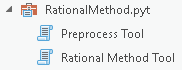# Quiz: The rational method toolbox

Institute for Environmental and Spatial Analysis...University of North Georgia

## 1   Overview

We want to refactor the Rational Method toolbox because its current workflow is not efficient when we study multiple watersheds. First, we want to remove the mask parameter to be able to handle multiple watersheds without having to guess the overall extent of the watersheds. Second, we want to combine runoff coefficient computation and flow accumulation processes into one tool called `PreprocessTool`. The idea is to create non-masked intermediate outputs using the Preprocess tool and use the Rational Method tool to process individual watersheds. Use test_data.zip for testing and validation. Check q2001.txt for final output.

## 2   Screenshots## 3   Penalties

Upload FirstLastname`_RationalMethod.pyt` to D2L ⇒ Assignments ⇒ Quiz 3 folder.

Debugging: -2 per line added, removed, or edited up to max points per question

Filenames and class names: -2 each

## 4   Question 1: Remove an unused parameter from the Rational Method tool

10 points

The `facc` parameter in the Rational Method tool is not used. Remove this parameter from `getParameterInfo()` and `execute()`.

## 6   Question 3: Combine runoff coefficient computation and flow accumulation

30 points

Move runoff coefficient computation from the Rational Method tool to the Flow Accumulation tool and rename the Flow Accumulation tool to the Preprocess tool. Its class name should be `PreprocessTool`. Remember, we don’t want to apply any mask using the ExtractByMask tool.

## 7   Question 4: Add a new required input parameter for the runoff coefficient raster to the Rational Method tool

10 points

Since we moved runoff coefficient calculation to the Preprocess tool, the Rational Method tool needs to take a runoff coefficient raster as an input. Add a new required input parameter called `runoff_coef` for the runoff coefficient raster to `getParameterInfo()` in the Rational Method tool.

## 8   Question 5: Apply output watersheds as a mask in the Rational Method tool

20 points

Extract the `runoff_coef` raster by output watersheds (`wsheds_ras`) before the cell area calculation where the `runoff_coef_ras` raster object is first used. Note that the input `runoff_coef` raster is not clipped to the watershed boundary while the `runoff_coef_ras` raster is.

## 9   Question 6: Calculate the total of output watersheds

20 points

Since we use the `OBJECTID` field in the pencil point layer (`outlets`), watershed IDs or cell values in the `wsheds_ras` are greater than 0. Use the following code snippet to add the total area of output watersheds in square miles to the output file.

``````# grab the raster object
wsheds_arr = arcpy.RasterToNumPyArray(wsheds_ras, nodata_to_value=0)

# count non-zero cells
wsheds_cells = sum(sum(wsheds_arr > 0))

# calculate the total area of watersheds in square miles
wsheds_area = wsheds_cells * YOUR_CODE``````

Remember that not all DEMs are in the same linear unit. Also, don’t forget to write the following line to the output file:

`Area of Watersheds = {wsheds_area} sqmi`

## 10   Bonus question: Reorder and relabel the parameters

10 points

Reorder and relabel the parameters in both tools to have exactly the same interface as the screenshots.